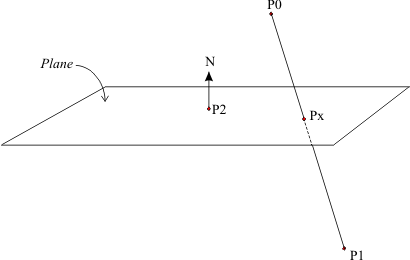Wednesday February 1, 2023
 home | about | contact | DonationsThe Maths of 3D You can't have 3D without a little maths...
 p {margin-top:0; margin-bottom:0;} Line with Plane Intersection Tutorial by bkenwright@xbdev.netWe'll brake up our solution finding, into simple steps....its the way that I learned it, and I think its easier to follow this way.   o Step 1. Equation of our Line          P(t) = P0 + t(P1 - P0)   This equation is also popularly known as a parametric line equation. where     P0 is the starting point of our line     P1 is the end point of our line     t is variable which goes from 0 to 1, and is used to derive a position at any point along our line.   o Step 2. Equation for our Plane        N dot (Px - P2) = 0   where     Px is the point of intersection of our line     P2 is any point on the plane     N the normal for our plane This equation is quit logical when you think about it, as when N and (Px-P2) are at 90 degrees to each other.  Remember (Px-P2) is the vector from P2 to our intersection point.   o Step 3. Substitute 1 into 2.          N dot (Px - P2) = 0        N dot { P0+t(P1-P0) - P2 } = 0        N dot { P0+t(P1-P0) } - N dot (P2) = 0        N dot { P0+t(P1-P0) } = N dot (P2)        N dot (P0) + N dot { t(P1-P0) } = N dot (P2)        N dot { t(P1-P0) } = N dot { P2-P0 }    t is a constant so         t * N dot { P1-P0 } = N dot { P2-P0 }         t =  N dot {P2-P0} /  N dot {P1-P0}     o Step 4. We can substitute t back into our Line Equation to find the Point of intersection Px.        P(t) = P0 + t(P1 - P0)           Px = P0   +   [ N dot {P2-P0} /  N dot {P1-P0}]  *   (P1 - P0)         Notes. We should test for our line being perpendicular to the plane at the start, which usually means testing if  Ndot(P1-P0)==0 , which tells us that our line is never going to cross our plane.   t > 0 and t < 1 :  The intersection occurs between the two end points t = 0               :   The intersection falls on the first end point t = 1               :   Intersection falls on the second end point t > 1               :   Intersection occurs beyond second end Point t < 0               :   Intersection happens before 1st end point.

 Visitor: 9534626 { 229.27.38.75 } Copyright (c) 2002-2020 xbdev.net - All rights reserved. Designated articles, tutorials and software are the property of their respective owners.